telescopeѲptics.net          ▪▪▪▪                                             CONTENTS

10.2.4.5. Houghton corrector: secondary spectrum reduction

As the ray plots clearly show, chromatism of the aplanatic (symmetrical) single-glass Houghton corrector becomes unacceptably large as the mirror relative aperture approaches and exceeds ~ƒ/3. While both, secondary spectrum and spherochromatism contribute to it, it is the former that dominates. The cause of the Houghton secondary spectrum is that its lens elements are not ideal thin lenses with near-zero thickness and separation. Consequently, the thin lens focal length formula given by (1/ƒ)=(1/ƒ1)+(1/ƒ2) doesn't apply. Instead, the effective focal length is given by the thick lens focal length formula for a pair of lenses,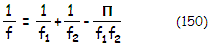with the individual focal lengths of the front and rear lens, as the actual thick-lens focal lengths, given by: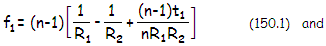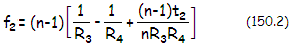respectively, with the quantity Π being the separation between the second principal plane of the front lens and the first principal plane of the rear lens (FIG. 202) given by: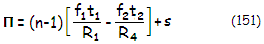with ƒ1=(n-1)[(1/R1)-1/R2] and ƒ2=(n-1)[(1/R3)-1/R4] being the front and rear lens focal length, respectively, t1 and t2 the respective lens center thicknesses, s the lens separation and R1, R2, R3 and R4 the respective lens surface radii. With 1/ƒ1 and 1/ƒ2 being very close numerically, it is the value of Π that mainly determines corrector's power (Eq. 150), and hence its secondary spectrum.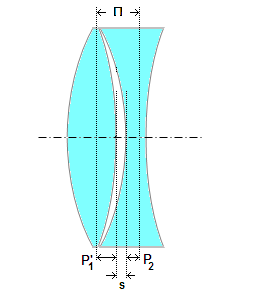FIGURE 202: Houghton corrector principal plane separation Π, between the second principal plane of the front lens (P1') and the first principal plane of the second lens (P2), is the main determinant of the effective non-zero focal length of the doublet. According to Eq. 151, the principal plane separation increases with lens thickness and lens spacing (s), reducing further corrector (positive) focal length, nearly in proportion to ~ƒ1ƒ2/Π. This in turn results in the increase of corrector's secondary spectrum. The principal plane separation - and secondary spectrum - for given aperture also increase with more strongly curved lens surfaces, needed to correct spherical aberration and coma of faster mirrors. When the two lenses are in contact, the separation s is likely to be, roughly, somewhat less than a half of the value of Π. This means that any relative increase in lens separation will result in less than half as much of a relative increase of secondary spectrum. With the typical values of ƒ1/R1~1.3 and ƒ2/R4~0.5, change in the thickness of the front lens results in secondary spectrum larger by a factor of ~2.5 than with identical thickness increase of the rear lens. Also, any relative change in the front lens thickness will result in ~1.3 times greater secondary spectrum than identical increase in lens separation, while thickness increase of the rear lens will result in the increase of secondary spectrum smaller by a factor of 0.5 than identical increase in lens separation.

As can be seen from Eq. 151, the size of Π it is not affected by a change in index, or radii, but it does change in proportion to lens thickness. Since the lenses can only be so thin, there is a practical minimum level of secondary spectrum that can not be further lessened with a single-glass Houghton corrector. It could only be cancelled by changing the sign of R4, but it would require the rear element to be a negative out-curving meniscus, which would make corrector with acceptable coma impossible.

In the symmetrical corrector configuration, the positive element has slightly less power than the negative element as a single lens, but when combined the two have a weak positive power. Neglecting the smaller (negative) value of (1/ƒ1)-1/ƒ2 in Eq. 150, the corrector focal length is approximated by ƒc~ƒ1ƒ2/Π (at the end of this section is explained in more details why taking somewhat smaller value is likely to give better end result), with the variation in the focal length for non-optimized wavelengths approximated by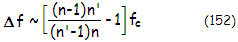where n and n' are the refractive index of the optimized and non-optimized wavelength, respectively. It places the secondary spectrum of the Houghton corrector - as the axial separation of its paraxial foci - roughly at ~ƒc/200, or some 10 times greater than in a doublet achromat. It is only due to the weak corrector's power that it induces relatively small amount of chromatism. Nominally, the secondary spectrum is negative for shorter (than the optimized) wavelengths, and positive for longer wavelengths - a consequence of the positive effective power of the corrector. It simply means that the former focus shorter, and the latter longer than the optimized wavelength.

Should the secondary spectrum be the only chromatic aberration present, the transverse chromatic blur diameter in units of the green e-line Airy disc diameter would be approximated by B~745(Δƒ)/Fc2, with Fc being the corrector's focal ratio, approximated by Fc~ƒc/D~ƒ1ƒ2/ΠD. In reality, secondary spectrum is always combined with a certain amount of sphero-chromatism, in which case the actual secondary spectrum is measured by the separation between best aberrated foci for different wavelengths, and the blur size results from the combined size of defocus (secondary spectrum) and spherical aberration (sphero-chromatism) for the wavelength.

Houghton corrector secondary spectrum can be minimized by either slightly weakening chromatic power of the front lens, or by slightly strengthening the rear lens. The two options for reducing the secondary spectrum of the Houghton corrector are: (1) abandoning symmetrical radii, and (2) using two different glass types for the lenses. Another option is to use plano-symmetrical corrector type, which has generally less of the residual power, hence smaller secondary spectrum. Abandoning equal radii design is a practical disadvantage fabrication-wise (also requiring more complex calculations), while plano-lens design puts constraints on coma-correction. Thus, the most effective way of reducing the Houghton secondary spectrum is by using two different glass types.

Starting out with a typical single-glass aplanatic Houghton corrector, minimizing the secondary spectrum requires small change in either power, or dispersion (or both) of one of the two lens elements. Lens power changes with (n-1), and its dispersion with 1/V, n being the glass refractive index and V its nominal dispersion. The measure of needed chromatic power change can be obtained from the general rule of achromatism for near-contact or contact doublet, requiring ƒ1/ƒ2=V2/V1. Since the aplanatic Houghton doublet acts as a thin lens pair in which the rear lens focal length is somewhat longer than that of the front lens, resulting in a weak positive power of the doublet, the appropriate dispersion V2 for minimizing the secondary spectrum should be different in approximately the same proportion.

Assuming thin-lens doublet, the rear lens effective focal length ƒ2e is obtained from 1/ƒc=(1/ƒ1)+1/ƒ2e, as 1/ƒ2e=1/ƒc-(1/ƒ1). The appropriate rear lens dispersion V2 can be obtained from ƒ1/ƒ2e=V2/V0, with V0 being the dispersion of the basic single-glass corrector. However, glass of different dispersion will almost invariably also have different refractive index. Since it is also a factor affecting chromatic power (secondary spectrum), it needs to be taken into account. With it, the achromatic relation takes the form ƒ1/ƒ2e=(n0-1)V2/(n2-1)V0, with needed properties of the achromatizing glass obtained from: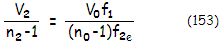with n0, V0 and n2, V2 being the refractive index (optimized wavelength) and Abbe number for the starting single-glass aplanatic corrector, and the achromatized negative element, respectively.

Helpful indicator of the effect of new glass type on secondary spectrum is given by 1/ç=(n2-1)V0/(n0-1)V2. This parameter can be called corrector's relative chromatic power. If it is the front lens to be achromatized, ç will be slightly smaller, and for the rear (negative) lens slightly greater than 1 (rough average with n~1.5 and mirrors in the ƒ/2.5-ƒ/3 range is ±1%). It reflects needed change in the relative chromatic power of one of the lens elements for near-optimal balancing of the combined secondary spectrum of the corrector.

In general, the second glass alternative for minimizing the secondary spectrum will be of very similar index and  dispersion values to those of the single-glass corrector to be achromatized (as the nominal dispersion and index change in opposite directions with significant index changes, it becomes difficult to impossible to find a glass with relative chromatism ç close to 1). Once suitable glass is found, it will make possible significant reduction of the secondary spectrum. An integral part of the optimization is tailoring out best combination of the secondary spectrum and spherical aberration, with lens thickness and separation also being possible factors (FIG. 203).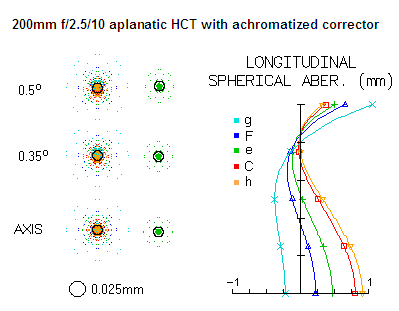FIGURE 203: Effect of achromatizing on the chromatism of a HCT with symmetrical aplanatic Houghton corrector (compare with Fig. 132a and 133a). By replacing the BK7 negative lens element glass with BK8, the wavefront error is reduced from 1.3 and 0.38 to 0.45 and 0.048 wave RMS for the h and r spectral lines, respectively (system a in the Appendix). By increasing lens separation, the chromatism is further reduced and optimized (balanced) to 0.14 and 0.12 wave RMS for the h and r lines, respectively, as shown on the plot (system b in the Appendix). The h-line correction is now nearly twice better than in non-achromatized plano-lens version, or at the level of a 4" ƒ/200 achromat. While still approximately double the chromatism of a comparable SCT, it is vastly improved over the non-achromatized version (note that further reduction could be possible). An ƒ/2.5 relative aperture for this aperture size is probably near-limit for all-spherical Houghton system. At this primary focal ratio, higher-order spherical, responsible for most of the scattered rays, cannot be corrected significantly better than 1/30 wave RMS without adding aspheric surface term.      SPEC'S

Achromatizing is still worthwhile at ~ƒ/3 mirror focal ratios. By switching a rear lens from BK7 to PK3, and increasing lens spacing from 1.4mm to 3mm (to compensate for the induced spherical aberration) in the ƒ/3/10 symmetrical aplanatic Houghton (Fig. 133c), corrector's chromatism is reduced from 0.37 and 0.1 to 0.085 and 0.043 wave RMS wavefront error for the violet h-line and red r-line, respectively. That compares very favorably to the reduction of chromatism by compromising it with some coma (0.28 and 0.075 wave RMS for the h- and r-lines, respectively). Note that the corrector type with all four radii different allows for still better color correction in a single-glass arrangement.

An alternative to increasing lens separation of the achromatized corrector is slightly (typically less than 1%) relaxing second radius. It is more likely to bring best foci of different wavelengths closer together, but the choice is best made using ray-trace.

As mentioned, secondary spectrum is main, but not a lone contributor to the chromatism of the Houghton corrector. The other is sphero-chromatism. As a consequence, the secondary spectrum - and chromatism in general - is minimized by bringing together best foci for different wavelengths, not the paraxial foci. This would certainly require more involved optimization than the one outlined above. In general, however, considering typical symmetrical aplanatic Houghton LA properties, even this crude form of optimization alone - in particular with the change in relative chromatic power purposely reduced to ~2/3 of the power differential indicated by the  ç value - should result in a significant reduction of corrector's chromatism.

The achromatizing glass often will, to some extent, also change the spherochromatism of the basic (single-glass) corrector. It can be for better, or for worse, but the effect is, in general, secondary to that of the change in size of secondary spectrum.